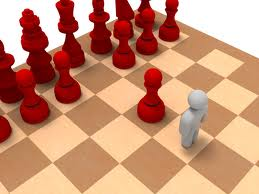# Count it.....

Probability Level 3What is the sum of total number of squares and rectangles on a $11 \times 11$ chessboard ?

Details

Since rectangles are squares, you are not supposed to double count the squares.

×